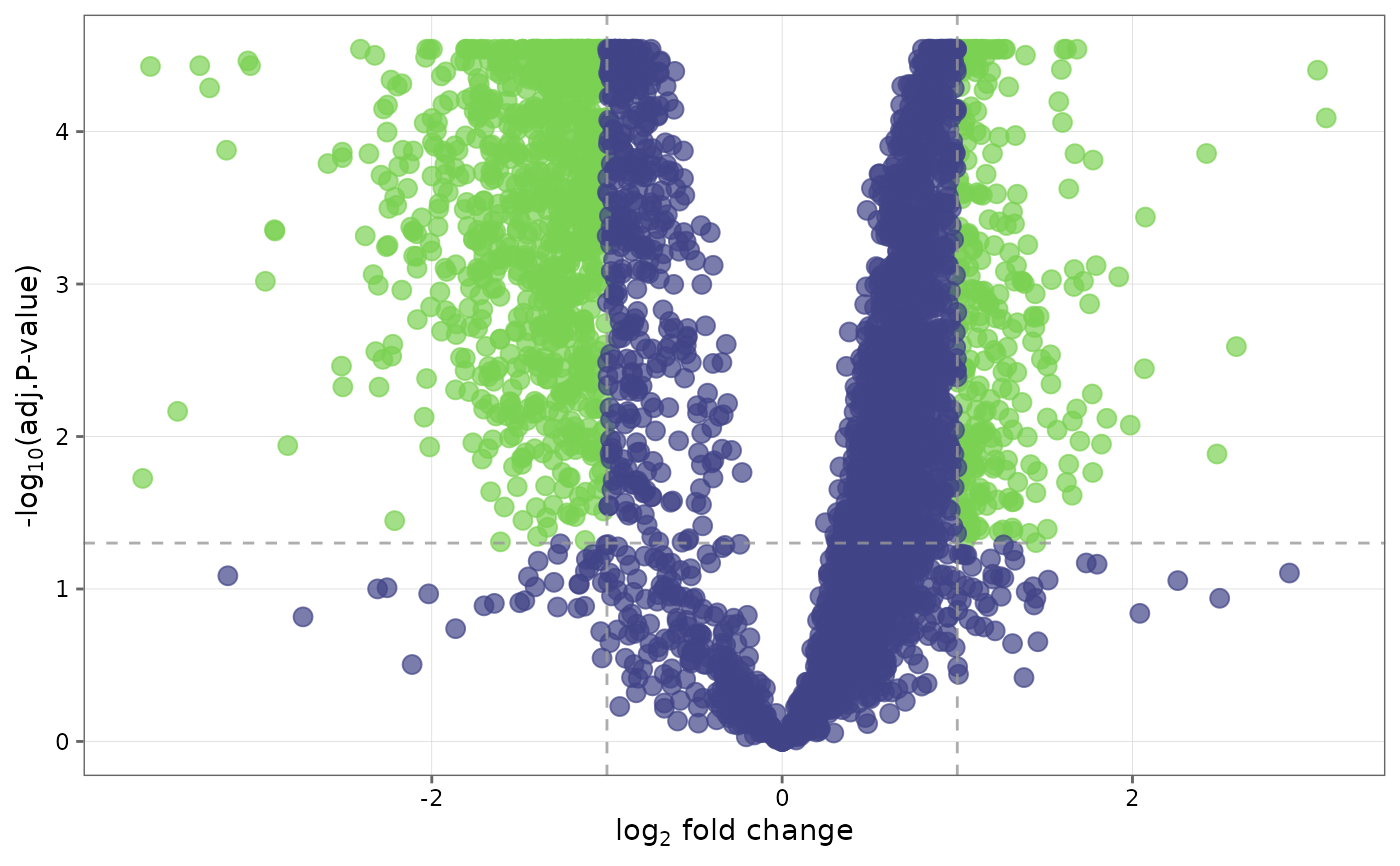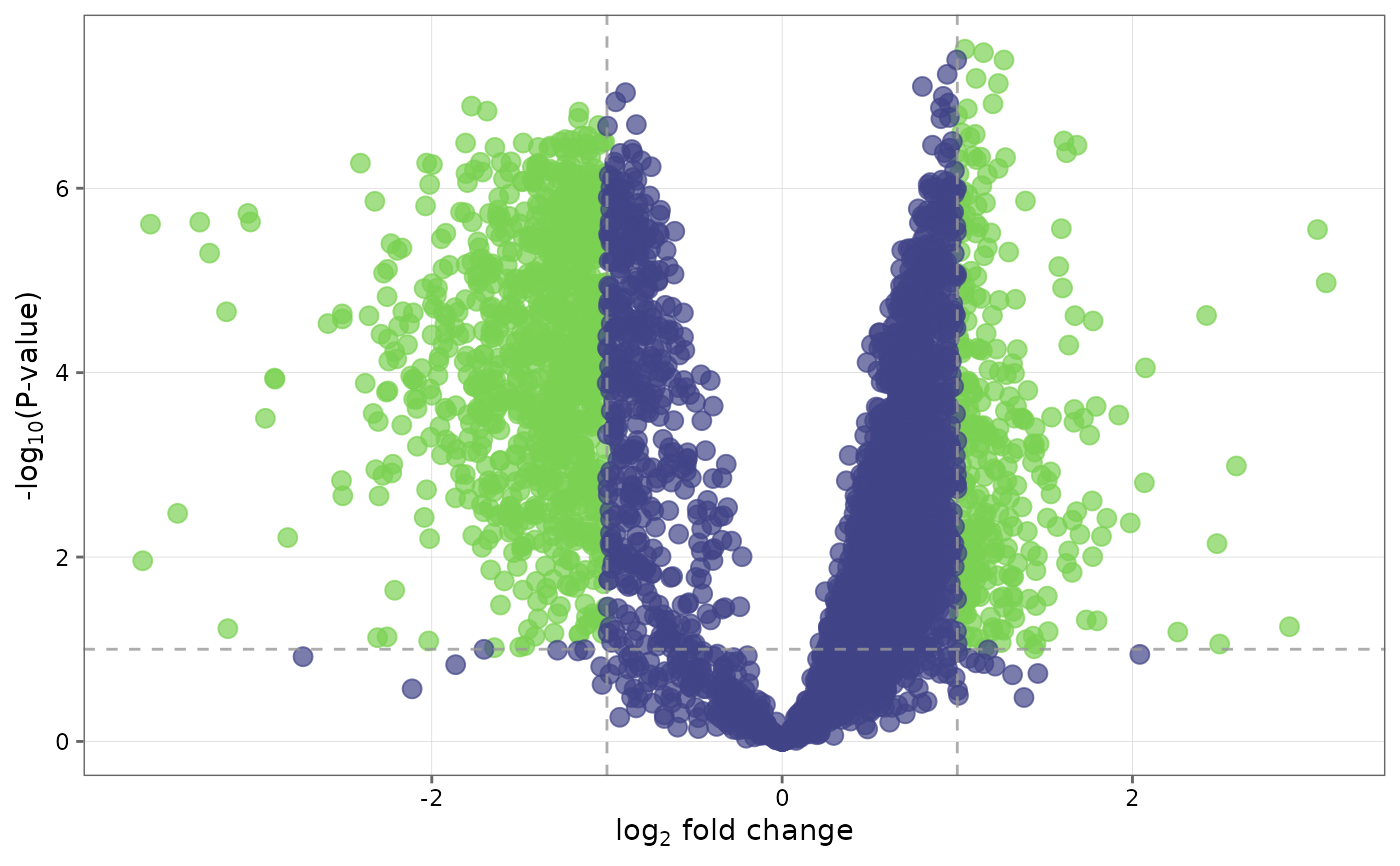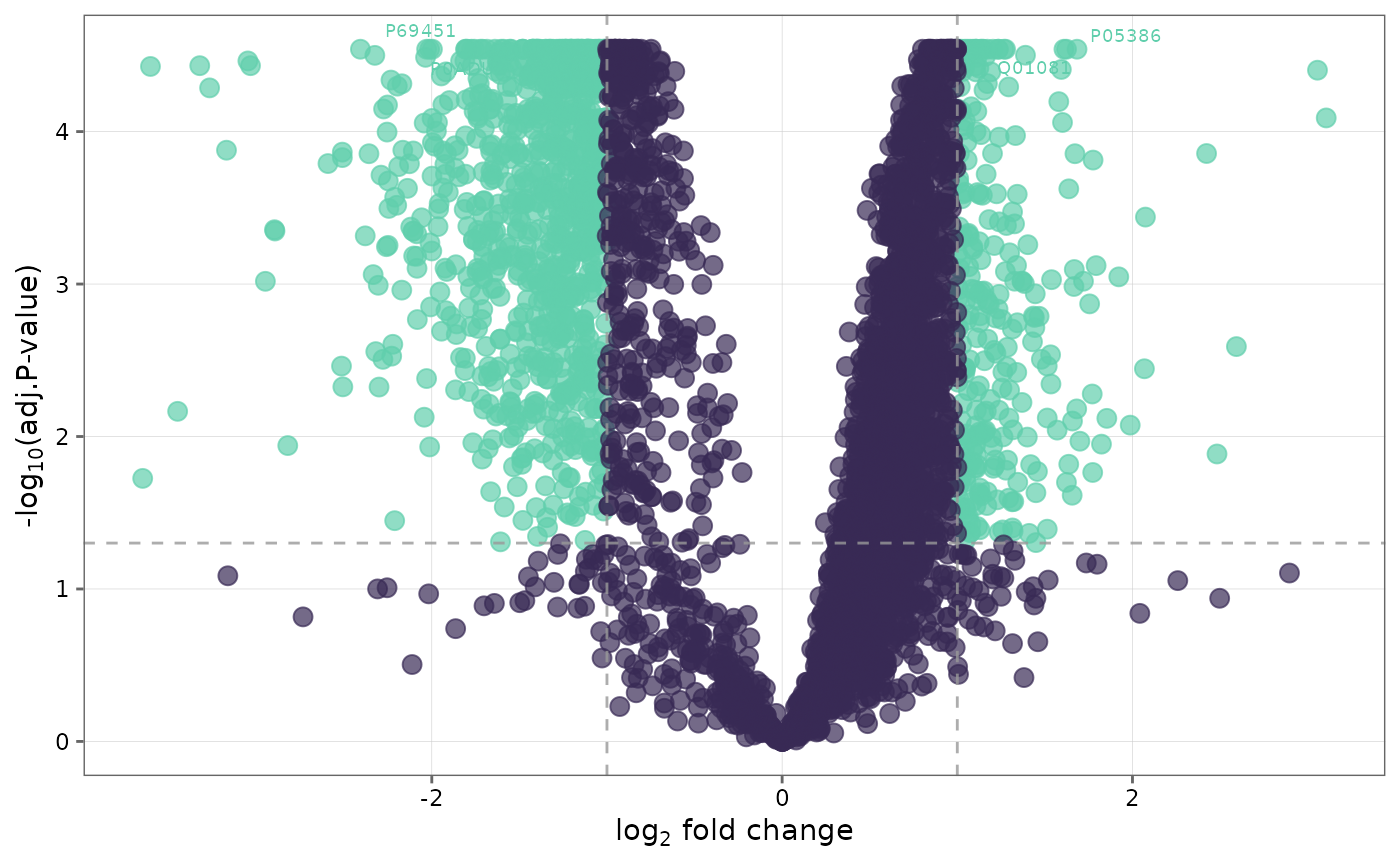This function generates volcano plots to visualize differentially expressed proteins between groups.

## Usage

volcano_plot(
fit_df,
cutoff = 0.05,
lfc = 1,
line_fc = TRUE,
line_p = TRUE,
palette = "viridis",
text_size = 10,
label_top = FALSE,
n_top = 10,
save = FALSE,
file_path = NULL,
file_name = "Volcano_plot",
file_type = "pdf",
plot_height = 7,
plot_width = 7,
dpi = 80
)

## Arguments

fit_df

A fit_df object from performing find_dep.

Method used for adjusting the p-values for multiple testing. Default is "BH".

sig

Criteria to denote significance. Choices are "adjP" (default) for adjusted p-value or "P" for p-value.

cutoff

Cutoff value for p-values and adjusted p-values. Default is 0.05.

lfc

Minimum absolute log2-fold change to use as threshold for differential expression.

line_fc

Logical. If TRUE(default), a dotted line will be shown to indicate the lfc threshold in the plot.

line_p

Logical. If TRUE(default), a dotted line will be shown to indicate the p-value cutoff.

palette

Viridis color palette option for plots. Default is "viridis". See viridis for available options.

text_size

Text size for axis text, labels etc.

label_top

Logical. If TRUE (default), labels are added to the dots to indicate protein names.

n_top

The number of top hits to label with protein name when label_top = TRUE. Default is 10.

save

Logical. If TRUE saves a copy of the plot in the directory provided in file_path.

file_path

A string containing the directory path to save the file.

file_name

File name to save the plot. Default is "Volcano_plot."

file_type

File type to save the plot. Default is "pdf".

plot_height

Height of the plot. Default is 7.

plot_width

Width of the plot. Default is 7.

dpi

Plot resolution. Default is 80.

## Value

A ggplot2 plot object.

## Details

• volcano_plot requires a fit_df object from performing differential expression analysis with find_dep.

• User has the option to choose criteria that denote significance.

• find_dep

• topTable and lmFit functions from the limma package.

## Author

Chathurani Ranathunge

## Examples


## Create a volcano plot with default settings.
volcano_plot(ecoli_fit_df)## Change significance criteria and cutoff
volcano_plot(ecoli_fit_df, cutoff = 0.1, sig = "P")## Label top 30 differentially expressed proteins and
## change the color palette of the plot
volcano_plot(ecoli_fit_df, label_top = TRUE, n_top = 30, palette = "mako")
#> Warning: ggrepel: 12 unlabeled data points (too many overlaps). Consider increasing max.overlaps